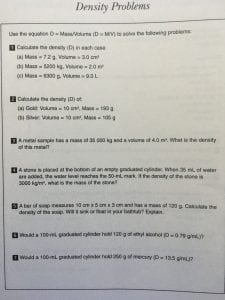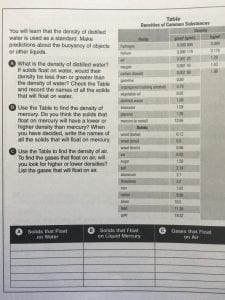Gr. 8 Fluids

# Gr. 8 Fluids

All of our learning, links, and information on Fluids can be found here. All student homework should be saved to your Science: Fluids folder in your google drive.

Introduction to Fluids

7.1 – Introduction to Fluids (read and answer question # 1) Due Mon. Dec. 7

7.2 – Characteristics of Fluids (read and answer questions #1, 3, 5 ab, 6) Due Mon. Dec. 7

Laminar vs. Tubulent Flow example

Viscosity

7.3 – Flow Rate and Viscosity (read and answer question # 1,2,3, 5) – Due Mon. Dec. 7

Calculating Flow Rates Worksheet (Make a copy and answer the 2 questions) – Due Mon. Dec. 7

Density

8.1 – Weight, Mass, and Volume (read and answer questions #2 & 3) – Due Mon. Dec. 7

8.3 – Density (read and answer questions # 2 -5) – Due Wed. Dec 9

What is Density and How to Calculate Student Note (Make a copy and add to your Fluids folder)

Calculating Density Worksheet # 1 (Make a copy and add to your Fluids folder before beginning)

Calculating Density Worksheet #2  (Pics below)

Worksheet #1 and #2 SolutionsAt Home Density Lab link (refer to lab report rubric for help on writing your conclusion)

Buoyancy and DisplacementFluids Under Pressure

9.1 – Putting the Squeeze on Fluids (Read and answer questions #1-4) Due Wed. Jan. 13th

9.4 – Effects of External Pressure on Fluids (Read, note the definitions of Pressure and Pascal’s Law, complete the ‘Try This’ section at home over a sink or bathtub)